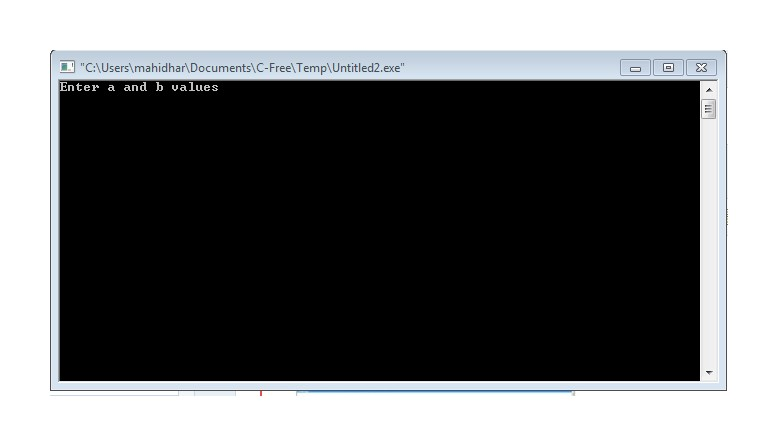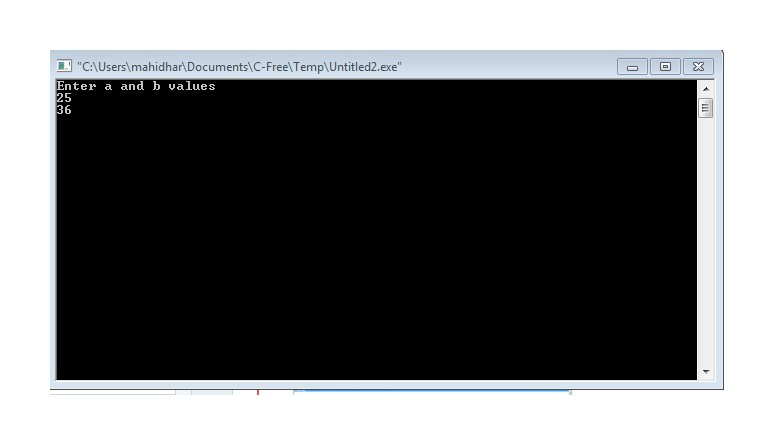## fb popup

/* remove if it's exist in your template */

### Printf and Scanf statements

PRINTF :-Used to print a given statement or a value.
SCANF:-Used to take the input at run time.

Before going to syntax and all we required to know about
Format Specifiers:

```%c            -character

%s            -string(array of characters).

%d or %i       -integer

%f             -float

%lf            -double

%Lf           -long double

%ld           -long int

%o            - octal value.

%u            -unsigned int

%lu           -unsigned long int.

%x            - hex value.```

```syntax for printf :-

To print a statement :-  printf("statement");

To print a value :- printf("%d",a);

Ex:-To print as :- a and b are 25 and 36

program:-

#include

main()

{

int a,b;

a=25;

b=36;

printf("a and b are %d and %d",a,b);

}```

```Syntax for scanf:-

To take an integer input:-scanf("%d",&a);

To take 2 a float value      :-scanf("%f%f",&a,&b);

and so on same for every format specifier.

Ex:-To print the same as above but taking values at runtime.

#include

main()

{

int a,b;

printf("Enter a and b values\n");

scanf("%d%d",&a,&b);

printf("a and b are %d and %d",a,b);

}

program and it's output:-output :-

First it asks us to enter a and b :-Then we enter the values as 25 and 36Then on pressing enter we get;-Here actually the compiler wont ask us to enter a and b values but it is we who are writing a printf statement to print a statement asking the user to "enter a and b values".

This is because we are the one who is writing a program and we know when to enter a value but if one of our friends came to us and compile the program,he might not know when to enter a value so, for him we should show when to enter and how many values are to be entered.

So,do not confuse about that particular statement.```

Different ways to print a decimal value and take value at runtime:
```Ex:-

1.)using %value d in scanf                                                                                                                                   Syntax:-scanf("%2d",&a); ------------It takes only first 2 digits of a value.

for example if we give 987 then it takes only 98 as input.

similarly for scanf("%3d",&a);-----------It takes only first 3 digits of a value,

for example if we give 98767 then it takes only 987 as input.```
```Ex:-

1.)using % .value d in printf

Syntax:-printf("%.2f",&a); ------------If the input is 1234.1234 then it gives only first 2 decimals in the value as 1234.12

similarly for scanf("%.3f",&a);-----------If the input is 1234.1234 then it gives only first 3 decimals in the value as 1234.123

Program:-```
` `
Output:-